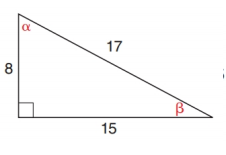Chapter 11.2, Problem 2E### Elementary Geometry for College St...

6th Edition
Daniel C. Alexander + 1 other
ISBN: 9781285195698

#### Solutions

Chapter
Section### Elementary Geometry for College St...

6th Edition
Daniel C. Alexander + 1 other
ISBN: 9781285195698
Textbook Problem
1 views

# In Exercises 1 to 6, find cos α and cos β .To determine

To find:

cosα and cosβ.

Explanation

Consider the following figure,

General formula for cosine ratio is given below,

From the given figure the value 17 is the hypotenuse value since it is opposite to right angle.

Then 8 is the length of the leg adjacent to α and 15 is the length of leg adjacent to β

### Still sussing out bartleby?

Check out a sample textbook solution.

See a sample solution

#### The Solution to Your Study Problems

Bartleby provides explanations to thousands of textbook problems written by our experts, many with advanced degrees!

Get Started

#### Solve the equations in Exercises 126. x4x2=6

Finite Mathematics and Applied Calculus (MindTap Course List)

#### ∫x−4dx = −4x−5 + C

Study Guide for Stewart's Single Variable Calculus: Early Transcendentals, 8th

#### Identify the basic features of a between-subjects research design.

Research Methods for the Behavioral Sciences (MindTap Course List)